垃圾邮件 机器学习_机器学习 垃圾邮件 - CSDN
• 垃圾邮件分类，作为初学者实践文本分类是一个不错的开始。文章将通过传统机器学习和深度学习的方法来解决分类问题。 机器学习方法：朴素贝叶斯、SVM、逻辑回归、RF、XGBoost、LightGBM 深度学习方法：
文章目录数据集格式清洗数据集- 去掉停用词- 去掉URL- 去掉HTML标签- 去掉表情符号- 去掉特殊符号使用机器学习的方法朴素贝叶斯、SVM1.1 读取数据1.2 构造词频矩阵1.3 训练并预测1.4 利用其它特征(TF-IDF)逻辑回归(LR)随机森林(RF)XGBoostLightGBM总结
垃圾邮件分类，作为初学者实践文本分类是一个不错的开始。文章将通过传统机器学习和深度学习的方法来解决分类问题。
机器学习方法：朴素贝叶斯、SVM、逻辑回归、RF、XGBoost、LightGBM
深度学习方法：垃圾邮件分类的各种尝试(深度学习篇)

开源代码地址（欢迎star~）：https://github.com/ljx02/Spam_Email_Classificaton
数据集下载链接：由于数据较小，暂时也放到了Git项目中

解决这个问题通用的思路是：

读取文本数据，包括训练集、测试集、验证集，如果数据质量不高考虑先清洗一下数据
创建词典，这一步有点抽象，可以看作是对语料中的词进行统计，方便后续提取特征
特征提取，通过对特征进行编码（向量化）
选择模型，开始训练分类器
验证模型，预测结果

数据集格式
总的数据集一共有4458条数据，将按照8:2进行划分训练集和验证集。通过分析发现，其中pam的数量有3866条，占数据集的大多数，可以考虑不平衡样本采样进行训练。
数据集的格式如图所示，有三列分别是ID，Label(pam、spam)，Email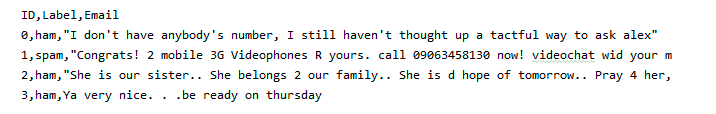清洗数据集
在实际中清洗数据也是非常必要的，套用一句俗话“数据决定了模型的上限”。常用的清洗数据的方法有：去掉停用词、去掉URL、去掉HTML标签、去掉特殊符号、去掉表情符号、去掉长重复字、将缩写补全、去掉单字、提取词干等等。当然，清洗数据也可能使模型变差，需要三思。提供部分处理的参考代码如下：
- 去掉停用词
from nltk.corpus import stopwords
stop = set(stopwords.words('english'))

text = "their are so many picture. how are you do this time very much!"
clean_text = []
for word in word_tokenize(text):
if word not in stop:
clean_text.append(word)
print(clean_text)

- 去掉URL
# 删除URL
example = "New competition launched :https://www.kaggle.com/c/nlp-getting-started"
def remove_URL(text):
url = re.compile(r'https?://\S+|www\.\S+')
return url.sub(r'', text)

print(remove_URL(example))

- 去掉HTML标签
# 删除HTML标签
example = """<div>
<h1>Real or Fake</h1>
<p>Kaggle </p>
<a href="https://www.kaggle.com/c/nlp-getting-started">getting started</a>
</div>"""
def remove_html(text):
html = re.compile(r'<.*?>')
return html.sub(r'', text)

- 去掉表情符号
# 删除表情符号
def remove_emoji(text):
emoji_pattern = re.compile("["
u"\U0001F600-\U0001F64F"  # emoticons
u"\U0001F300-\U0001F5FF"  # symbols & pictographs
u"\U0001F680-\U0001F6FF"  # transport & map symbols
u"\U0001F1E0-\U0001F1FF"  # flags (iOS)
u"\U00002702-\U000027B0"
u"\U000024C2-\U0001F251"
"]+", flags=re.UNICODE)
return emoji_pattern.sub(r'', text)

- 去掉特殊符号
import string
def remove_punct(text):
# 对punctuation中的词进行删除
table = str.maketrans('', '', string.punctuation)
return text.translate(table)

使用机器学习的方法
朴素贝叶斯、SVM
1.1 读取数据
import pandas as pd
# 读邮件数据CSV

1.2 构造词频矩阵
第二步同时也构造了词典，因为词频矩阵的行是所有文章中出现的词，纵轴代表文章。统计每一篇文章中出现相应词的次数。举个例子如下：
['Hello','How are you','Are you OK']


hello
how
are
you
…

0
1
0
0
0
…

1
0
1
1
1
…

2
0
0
1
1
…

代码实现，使用sklearn中的工具，进行计算得出词频矩阵。这里需要注意的是，在处理训练集时，使用fit_transform而在测试得时候使用transform，因为测试的时候是不需要再训练。
from sklearn.feature_extraction.text import CountVectorizer
# 将内容转为list类型
train_email = np.array(train_email).reshape((1, len(train_email))).tolist()
train_label = np.array(train_label).reshape((1, len(train_email))).tolist()
# 使用词袋模型
vectorizer = CountVectorizer()
# CountVectorizer类会把文本全部转换为小写，然后将文本词块化。主要是分词，分标点
data_train_cnt = vectorizer.fit_transform(data_train)
data_test_cnt = vectorizer.transform(data_dev)

1.3 训练并预测
from sklearn.naive_bayes import MultinomialNB
from sklearn.svm import LinearSVC
from sklearn.metrics import confusion_matrix
# 利用贝叶斯的方法
clf = MultinomialNB()
clf.fit(data_train_cnt, label_train)
score = clf.score(data_test_cnt, label_dev)
print(score)

# 利用SVM的方法
svm = LinearSVC()
svm.fit(data_train_cnt, label_train)
score = svm.score(data_test_cnt, label_dev)
print(score)

result_svm = svm.predict(data_test_cnt)
print(confusion_matrix(label_dev, result_svm))

运行结果如下：分数都不错，总的感觉贝叶斯效果稍好一点（当然数据有限~~）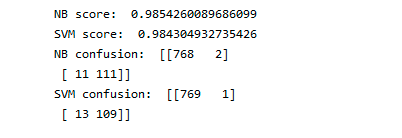1.4 利用其它特征(TF-IDF)
使用不同的特征进行训练，比较有名的是TF-IDF（词频-逆文档频率），逆文档频率含义是如果某个词或短语具有良好的类别区分能力，并且在其它文档中很少出现，则认为这个词或者短语有很好的类别区分能力，比较适合分类。通俗地讲，如果一个词在其中一个文档中出现过，在其它文章中没有出现过，则将这个词的权重增大。反之如果这个词大量出现在所有文档中，则表示这个词对于分类来说不是很重要，出现再多也无实际意义，所以降低其权重。计算逆文档频率一般采用下图公式：TF-IDF就是词频和逆文档频率的乘积。
具体代码如下（有两种写法）：
第一种：直接利用TfidfTransformer对词频矩阵进行计算，得出TF-IDF矩阵
from sklearn.feature_extraction.text import TfidfTransformer

vectorizer = CountVectorizer()
# CountVectorizer类会把文本全部转换为小写，然后将文本词块化。主要是分词，分标点
data_train_cnt = vectorizer.fit_transform(data_train)
transformer = TfidfTransformer()
data_train_tfidf = transformer.fit_transform(data_train_cnt)

data_test_cnt = vectorizer.transform(data_dev)
data_test_tfidf = transformer.transform(data_test_cnt)


第二种：利用TfidfVectorizer对data_train直接操作，得出TF-IDF矩阵（最终结果是一样的）
from sklearn.feature_extraction.text import TfidfTransformer
from sklearn.feature_extraction.text import TfidfVectorizer

vectorizer_tfidf = TfidfVectorizer(sublinear_tf=True)
data_train_tfidf = vectorizer_tfidf.fit_transform(data_train)

data_test_tfidf = vectorizer_tfidf.transform(data_dev)

从结果分析，引入TF-IDF特征后效果有一点点波动，算做一种尝试。
逻辑回归(LR)
逻辑回归是用途最广的分类算法，适用性很强。但是想充分利用好逻辑回归，就得不断的调整参数，直到合理为止。具体代码和上边的极其相似，需要引入下面这句话：
from sklearn.linear_model import LogisticRegression

随机森林(RF)
随机森林是一种以决策树为基础的一种更高级的算法。随机森林即可以用于回归也可以用于分类。随机森林从本质上看属于集成学习的一种，通过建立几个模型组合起来解决一个问题。往往随机森林的表现要优于单一的决策树。实现随机森林的代码与上边的类似，已经有库提供这个方法了，只需要引入下边代码：
from sklearn.ensemble import RandomForestClassifier

XGBoost
XGBoost也属于集成学习，高效地实现了GBDT算法并进行了算法和工程上的许多改进。属于boosting的一种。XGBoost算法的核心思想如下：

不断地添加树，每次添加都是要学习一个新的f(x)，目的是拟合上次的残差
每个叶子节点对应一个分数，最终只需要将对应的分数相加起来就得到样本的预测值。

XGBoost的一大优势就是，能够自动学习出缺失值的处理策略。同时使用了一阶导数和二阶导数，有利于梯度下降的更快更准。
当然XGBoost也有缺点：

每轮迭代时，都需要遍历整个训练数据多次。耗时且占用较大内存。
预排序方法的时间和空间的消耗都较大。

同样，代码只需要引入库如下：
import xgboost as xgb

LightGBM
LightGBM是一个梯度Boosting框架，使用基于决策树的学习方法。具有以下优势：

更快的训练效率
低内存的使用
更高的准确率
支持并行化学习
可以处理大规模数据

利用LightGBM进行训练，直接引库就好：
import lightgbm as lgb

总结
尝试了不同的方法，总体不难，代码好多都有了方便的库函数辅助。但是每一种方法如果想发挥出它的最大效果，就得不断尝试修改参数。为了快捷调参，可以使用网格调参，具体使用方法已经写入代码中，可以查看GridSearchCV的使用方法。修改参数的第一步就是认识方法中的所有参数，下边我分享一些参数介绍的文章链接：
LightGBM介绍及参数调优
XGBoost介绍及参数调优
LogisticRegression介绍及参数调优
由于训练数据较少，最终发现NB的效果最理想。其它方法估计在大的数据集上会表现好一点，需要后续试验验证。后续可以改进的方法有：

调整参数
模型融合
引入额外信息和特征


展开全文自然语言处理
• 以下是根据《机器学习：实用案例解析》一书中的案列写的，感觉结果并没有达到预期的状态，欢迎大家指出我其中理解错的地方，哈哈哈。 原始数据：SpamAssassin语料库，http://spamassain.apache.org/publiccorpus/...
以下是根据《机器学习：实用案例解析》一书中的案列写的，感觉结果并没有达到预期的状态，欢迎大家指出我其中理解错的地方，哈哈哈。
原始数据：SpamAssassin语料库，http://spamassain.apache.org/publiccorpus/
spam：垃圾邮件
easy ham：易识别的正常邮件
hard ham：不易识别的正常邮件

文本分类算法：朴素贝叶斯分类器（假设所有词之间频次统计相互独立）
a）明显更可能在垃圾邮件中出现的词；
b）明显更可能在非垃圾邮件中出现的词。
分类结果：
a）假设电子邮件是垃圾邮件，看到其具体内容的概率；
b）假设电子邮件不是垃圾邮件，看到相同内容的概率。
预测：通过邮件正文内容本身预测邮件的类别

思路：
a）原始邮件预处理：去除头部，保留邮件正文；
b） 构建特征词类别知识库，通过从邮件正文中抽取特证词来构建邮件分类器的特征集。

语料库构造：量化特证词频率——构造一个词项-文档矩阵（TDM）N*M的矩阵，[i，j]表示词项i在文档j中出现的次数c）构建分类器：

构建一个数据框来保存所有特征项在垃圾邮件中的条件概率；
能在已知观测特征的前提下计算出邮件是垃圾的概率；
根据有多少邮件包含这个特征词项来定义一封邮件是垃圾邮件的条件概率；

一、首先处理spam垃圾邮件：

#加载程序包
library(tm)

library(ggplot2)

#设置数据源路径

spam.path <- file.path("data", "spam")

spam2.path <- file.path("data", "spam_2")

easyham.path <- file.path("data", "easy_ham")

easyham2.path <- file.path("data", "easy_ham_2")

hardham.path <- file.path("data", "hard_ham")

hardham2.path <- file.path("data", "hard_ham_2")

# 读取文件正文内容
# 每份邮件包含头部和正文两个部分一般由第一个空行分割（协议规定）
#打开每个文件，找到空行，并将空行之后的文本返回一个字符串向量，就是空行之后的所有文本拼接之后字符串。
get.msg <- function(path)

{

con <- file(path, open = "rt", encoding = "latin1")

#读取每一行文本返回为字符串向量的一个元素

# 定位第一个空行，并抽取出其后的所有文本

# tryCatch来捕获异常，不输出,原先代码为msg <- text[seq(which(text == "") + 1, length(text), 1)] 报错

msg <- tryCatch(text[seq(which(text == "") + 1, length(text), 1)], error = function(e) e)

#关闭文件

close(con)

#paste把向量拼接成一个单条文本元素，用"\n"换行来分割各个元素

return(paste(msg, collapse = "\n"))

}

#保存邮件内容，使得向量的每个元素就是一封邮件内容。

#dir 获得路径下所有文件名列表
spam.docs <- dir(spam.path)

#读取文件名，cmds是用于移动文件的命令，不是数据集，要排除掉

#spam.docs 就是包含用于训练的所有文件名的一个字符向量

spam.docs <- spam.docs[which(spam.docs != "cmds")]

#sapply，对每个邮件应用get.msg函数，通过返回值构建一个文本向量

all.spam <- sapply(spam.docs,

function(p) get.msg(paste(spam.path, p,sep="")))

#检查每个向量元素的名称与文件名是否一一对应

#构建一个文本资料库

#定义get.td函数：输入邮件文本向量；输出TDM；

#tm包提供若干方法用于构建语料库；
get.tdm <- function(doc.vec)

{

#stopwords = TRUE,告诉tm在所有文本中移除488个最常见的英文停用词；

#查看停用列表stopwords()；

#removePunctuation = TRUE,移除标点符号；

#removeNumbers = TRUE,移除数字；

#minDocFreq = 2,只保留出现次数大于1次的词；

control <- list(stopwords = TRUE,

removePunctuation = TRUE,

removeNumbers = TRUE,

minDocFreq = 2)

#VectorSource函数构建source对象以利用邮件向量构建语料库.

doc.corpus <- Corpus(VectorSource(doc.vec))

doc.dtm <- TermDocumentMatrix(doc.corpus, control)

#返回的是TMD文本

return(doc.dtm)

}

spam.tdm <- get.tdm(all.spam)

#至此垃圾邮件处理完成，下面开始构建分类器

#用TDM构建一套垃圾邮件训练数据

#输入：TDM

#输出：词频与占比

#as.matrix，把TDM对象转换成R的标准矩阵；
spam.matrix <- as.matrix(spam.tdm)

#rowSums，创建一个向量，包含每个特征在所有文档中的总频次；

spam.counts <- rowSums(spam.matrix)

#data.frame，把一个字符向量和一个数值向量结合在一起；

#stringsAsFactors = FALSE，默认会转换成因子类型，所以要关闭；

spam.df <- data.frame(cbind(names(spam.counts),

as.numeric(spam.counts)),

stringsAsFactors = FALSE)

#修改列名

names(spam.df) <- c("term", "frequency")

#把频次转化成数值向量

spam.df$frequency <- as.numeric(spam.df$frequency)

#计算一个特定词项所出现的文档在所有文档中所占的比例；

#sapply，把每行的行号读入；

#function(i)，统计该行中值为正数的元素个数/TDM中列的总数（也就是垃圾邮件语料库中文档总数）；

spam.occurrence <- sapply(1:nrow(spam.matrix),

function(i)

{

length(which(spam.matrix[i, ] > 0)) / ncol(spam.matrix)

})

#统计整个垃圾邮件语料库中每个词项的出现概率；

spam.density <- spam.df$frequency / sum(spam.df$frequency)

# density: 统计整个语料库中每个词项的频次(不用此来分类, 如果想知道某些词是否影响结果,对比频次相当有用)

spam.df <- transform(spam.df,

density = spam.density,

occurrence = spam.occurrence)

# 查看统计结果

二、构建正常邮件的训练数据

与垃圾邮件一样，路径使用data/easy_ham

选取和垃圾邮件一样的数量500封。

# 易识别的正常邮件

easyham.docs <- dir(easyham.path)

easyham.docs <- easyham.docs[which(easyham.docs != "cmds")]

all.easyham <- sapply(easyham.docs[1:length(spam.docs)],

function(p) get.msg(file.path(easyham.path, p)))

easyham.tdm <- get.tdm(all.easyham)

easyham.matrix <- as.matrix(easyham.tdm)

easyham.counts <- rowSums(easyham.matrix)

easyham.df <- data.frame(cbind(names(easyham.counts),

as.numeric(easyham.counts)),

stringsAsFactors = FALSE)

names(easyham.df) <- c("term", "frequency")

easyham.df$frequency <- as.numeric(easyham.df$frequency)

easyham.occurrence <- sapply(1:nrow(easyham.matrix),

function(i)

{

length(which(easyham.matrix[i, ] > 0)) / ncol(easyham.matrix)

})

easyham.density <- easyham.df$frequency / sum(easyham.df$frequency)

easyham.df <- transform(easyham.df,

density = easyham.density,

occurrence = easyham.occurrence)

# 查看统计结果

词 频次 概率 文档占比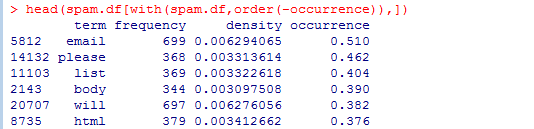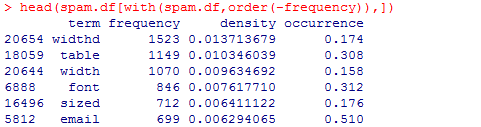若果用频次来作为垃圾邮件的训练数据，会把某些包含table的垃圾邮件权重调的太高，因为并 不是所有的垃圾邮件都是table方式生成的；

===所以用根据有多少邮件包含这个特征词来定义是垃圾邮件的概率，即occurrence；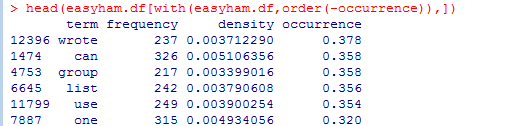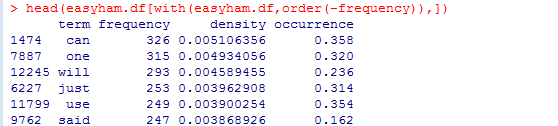分析：对比垃圾邮件和正常邮件可以发现，正常邮件的特征词占比与词频比较稀疏平均；

===如果一封邮件中包含有一两个与垃圾邮件非常相关的词，那么就需要很多非垃圾词才能将其分类为正常邮件。

三、定义分类器并用不易识别的正常邮件进行测试

思考：对于新的邮件中哪些在训练集中的词与不在训练集中的词该如何处理？

思路：计算各个词在训练集中的概率乘积；

对于已在的，直接计算条件概率；

对于未在的，根据某些分布给它们赋予一个随机概率；或者用自然语言处理（NLP）估计一个词 项在上下文中的“垃圾倾向”。

===给这些没出现在数据集中的词赋予一个特别小的概率0.0001%；

处理：每个类别的先验概率都默认50%（假设是垃圾邮件和正常邮件的可能性相同）；

对新的邮件抽取内容，统计词项。

# 定义一个分类器函数

# get.msg 抽取出邮件正文

# get.tdm 转换成TDM

# rowSums 计算特征词项的频率

classify.email <- function(path, training.df, prior = 0.5, c = 1e-6)

{

msg <- get.msg(path)

msg.tdm <- get.tdm(msg)

msg.freq <- rowSums(as.matrix(msg.tdm))

# intersect函数-寻找邮件特征词与训练集的交集

# msg.match-保存这封邮件中所有在训练集spam.df中出现过的特征词项

msg.match <- intersect(names(msg.freq), training.df$term) # 判断是否有词出现在训练集中，若有则计算属于训练集对应类别邮件的概率 # 空集返回prior * c ^ (length(msg.freq)) ，先验概率乘以小概率值c的邮件特征数次幂。由于没有可判断的词，所以这样的结果是被分为垃圾邮件概率很小； # 若不为空，match-查找词项在训练级的term列出现的位置，计算交集词的occurrence概率 if(length(msg.match) < 1) { return(prior * c ^ (length(msg.freq))) } else { match.probs <- training.df$occurrence[match(msg.match, training.df$term)] return(prior * prod(match.probs) * c ^ (length(msg.freq) - length(msg.match))) } } # 用不易识别的正常邮件进行测试 # 获取文件路劲，sapply封装对垃圾邮件和正常邮件的测试 hardham.docs <- dir(hardham.path) hardham.docs <- hardham.docs[which(hardham.docs != "cmds")] # hardham.spamtest 保存每封邮件在给定对应训练数据的前提下是垃圾邮件的条件概率计算结果 # hardham.hamtest 保存正常邮件的条件概率 hardham.spamtest <- sapply(hardham.docs, function(p) classify.email(file.path(hardham.path, p), training.df = spam.df)) hardham.hamtest <- sapply(hardham.docs, function(p) classify.email(file.path(hardham.path, p), training.df = easyham.df)) # 比较两个概率的大小 hardham.res <- ifelse(hardham.spamtest > hardham.hamtest, TRUE, FALSE) # 查看结果 summary(hardham.res) 四、用所有邮件类型测试分类器 # 定义一个函数用于一次性完成对所有邮件概率的比较 # 根据spam.df和easyham.df判定是否为垃圾邮件 spam.classifier <- function(path) { pr.spam <- classify.email(path, spam.df) pr.ham <- classify.email(path, easyham.df) return(c(pr.spam, pr.ham, ifelse(pr.spam > pr.ham, 1, 0))) } # 获取所有的邮件列表 easyham2.docs <- dir(easyham2.path) easyham2.docs <- easyham2.docs[which(easyham2.docs != "cmds")] hardham2.docs <- dir(hardham2.path) hardham2.docs <- hardham2.docs[which(hardham2.docs != "cmds")] spam2.docs <- dir(spam2.path) spam2.docs <- spam2.docs[which(spam2.docs != "cmds")] # 易于辨别的正常邮件 easyham2.class <- suppressWarnings(lapply(easyham2.docs, function(p) { spam.classifier(file.path(easyham2.path, p)) })) # 不易辨别的正常邮件 hardham2.class <- suppressWarnings(lapply(hardham2.docs, function(p) { spam.classifier(file.path(hardham2.path, p)) })) # 垃圾邮件 spam2.class <- suppressWarnings(lapply(spam2.docs, function(p) { spam.classifier(file.path(spam2.path, p)) })) # 创建数据框存放结果 easyham2.matrix <- do.call(rbind, easyham2.class) easyham2.final <- cbind(easyham2.matrix, "EASYHAM") hardham2.matrix <- do.call(rbind, hardham2.class) hardham2.final <- cbind(hardham2.matrix, "HARDHAM") spam2.matrix <- do.call(rbind, spam2.class) spam2.final <- cbind(spam2.matrix, "SPAM") class.matrix <- rbind(easyham2.final, hardham2.final, spam2.final) class.df <- data.frame(class.matrix, stringsAsFactors = FALSE) names(class.df) <- c("Pr.SPAM" ,"Pr.HAM", "Class", "Type") class.df$Pr.SPAM <- as.numeric(class.df$Pr.SPAM) class.df$Pr.HAM <- as.numeric(class.df$Pr.HAM) class.df$Class <- as.logical(as.numeric(class.df$Class)) class.df$Type <- as.factor(class.df$Type) # 查看结果 head(class.df)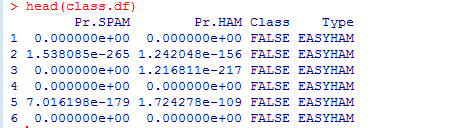# 统计识别率 get.results <- function(bool.vector) { results <- c(length(bool.vector[which(bool.vector == FALSE)]) / length(bool.vector), length(bool.vector[which(bool.vector == TRUE)]) / length(bool.vector)) return(results) } easyham2.col <- get.results(subset(class.df, Type == "EASYHAM")$Class)

hardham2.col <- get.results(subset(class.df, Type == "HARDHAM")$Class) spam2.col <- get.results(subset(class.df, Type == "SPAM")$Class)

class.res <- rbind(easyham2.col, hardham2.col, spam2.col)

colnames(class.res) <- c("NOT SPAM", "SPAM")

print(class.res)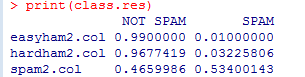邮件类型                 3045
正常邮件
垃圾邮件
易识别的正常邮件    1400
1386 （99%）
14（1%）
不易识别的正常邮件 248
240 （96.8%）
8 （3.2%）
垃圾邮件                  1397
651 （46.6%）
746 （53.4%）

分析：可以看出对于正常邮件，分类效果还不错，但是对与垃圾邮件，效果并不是很理想；

主要问题是许多垃圾邮件被判为正常邮件，在无法确定的情况下，程序判为正常邮件的概率大，说明垃圾邮件的训练数据不足，与垃圾邮件相关的特征没有被纳入。

五、改进

正常邮件中垃圾邮件和正常邮件的比例为2：8，首先尝试改进先验概率；

效果并不明显，即使先验概率改为1，也就是当无法判断的时候都判断为垃圾邮件，对垃圾邮件的分类效果依然不好，是否可能正常邮件训练集中的特征有问题，存在一些垃圾邮件和正常邮件中都会出现的，而原先的训练中没被垃圾邮件收入，而被正常邮件收录了的？？？（这是个人运行后存在的问题，还有待解决。）

最后附上完整的R代码

# 清空变量，加载程序包
rm(list = ls())
if(require(tm) == FALSE) {
install.packages("tm")
library(tm)
}
if(require(ggplot2) == FALSE) {
install.packages("ggplot2")
library(ggplot2)
}

# 设置数据源路劲
spam.path <- file.path("data", "spam")
spam2.path <- file.path("data", "spam_2")
easyham.path <- file.path("data", "easy_ham")
easyham2.path <- file.path("data", "easy_ham_2")
hardham.path <- file.path("data", "hard_ham")
hardham2.path <- file.path("data", "hard_ham_2")

# 首先处理垃圾邮件
# 定义函数，用于读取邮件正文内容，以第一个空行作为开始标志
get.msg <- function(path)
{
con <- file(path, open = "rt", encoding = "latin1")
msg <- tryCatch(text[seq(which(text == "") + 1, length(text), 1)], error = function(e) e)
close(con)
return(paste(msg, collapse = "\n"))
}

# 构建一个文本资料库
get.tdm <- function(doc.vec)
{
doc.corpus <- Corpus(VectorSource(doc.vec))
control <- list(stopwords = TRUE,
removePunctuation = TRUE,
removeNumbers = TRUE,
minDocFreq = 2)
doc.dtm <- TermDocumentMatrix(doc.corpus, control)
return(doc.dtm)
}

# 保存邮件内容
spam.docs <- dir(spam.path)
spam.docs <- spam.docs[which(spam.docs != "cmds")]
all.spam <- sapply(spam.docs,
function(p) get.msg(file.path(spam.path,p)))

# 获取文本资料库
spam.tdm <- get.tdm(all.spam)

# 用TDM构建一套垃圾邮件训练数据
spam.matrix <- as.matrix(spam.tdm)
spam.counts <- rowSums(spam.matrix)
spam.df <- data.frame(cbind(names(spam.counts),
as.numeric(spam.counts)),
stringsAsFactors = FALSE)
names(spam.df) <- c("term", "frequency")
spam.df$frequency <- as.numeric(spam.df$frequency)
spam.occurrence <- sapply(1:nrow(spam.matrix),
function(i)
{
length(which(spam.matrix[i, ] > 0)) / ncol(spam.matrix)
})
spam.density <- spam.df$frequency / sum(spam.df$frequency)

# 统计整个语料库中每个词项的频次
spam.df <- transform(spam.df,
density = spam.density,
occurrence = spam.occurrence)

# 易识别的正常邮件

easyham.docs <- dir(easyham.path)
easyham.docs <- easyham.docs[which(easyham.docs != "cmds")]
all.easyham <- sapply(easyham.docs[1:length(spam.docs)],
function(p) get.msg(file.path(easyham.path, p)))

easyham.tdm <- get.tdm(all.easyham)

easyham.matrix <- as.matrix(easyham.tdm)
easyham.counts <- rowSums(easyham.matrix)
easyham.df <- data.frame(cbind(names(easyham.counts),
as.numeric(easyham.counts)),
stringsAsFactors = FALSE)
names(easyham.df) <- c("term", "frequency")
easyham.df$frequency <- as.numeric(easyham.df$frequency)
easyham.occurrence <- sapply(1:nrow(easyham.matrix),
function(i)
{
length(which(easyham.matrix[i, ] > 0)) / ncol(easyham.matrix)
})
easyham.density <- easyham.df$frequency / sum(easyham.df$frequency)

easyham.df <- transform(easyham.df,
density = easyham.density,
occurrence = easyham.occurrence)

# 定义一个分类器函数
# 寻找邮件特征词与训练集的交集
classify.email <- function(path, training.df, prior = 0.8, c = 1e-6)
{
msg <- get.msg(path)
msg.tdm <- get.tdm(msg)
msg.freq <- rowSums(as.matrix(msg.tdm))
msg.match <- intersect(names(msg.freq), training.df$term) if(length(msg.match) < 1) { return(prior * c ^ (length(msg.freq))) } else { match.probs <- training.df$occurrence[match(msg.match, training.df$term)] return(prior * prod(match.probs) * c ^ (length(msg.freq) - length(msg.match))) } } # 用不易识别的正常邮件进行测试 hardham.docs <- dir(hardham.path) hardham.docs <- hardham.docs[which(hardham.docs != "cmds")] hardham.spamtest <- sapply(hardham.docs, function(p) classify.email(file.path(hardham.path, p), training.df = spam.df)) hardham.hamtest <- sapply(hardham.docs, function(p) classify.email(file.path(hardham.path, p), training.df = easyham.df)) hardham.res <- ifelse(hardham.spamtest > hardham.hamtest, TRUE, FALSE) summary(hardham.res) # 定义一个函数用于一次性完成对所有邮件概率的比较 spam.classifier <- function(path) { pr.spam <- classify.email(path, spam.df) pr.ham <- classify.email(path, easyham.df) return(c(pr.spam, pr.ham, ifelse(pr.spam > pr.ham, 1, 0))) } # 获取所有的邮件列表 easyham2.docs <- dir(easyham2.path) easyham2.docs <- easyham2.docs[which(easyham2.docs != "cmds")] hardham2.docs <- dir(hardham2.path) hardham2.docs <- hardham2.docs[which(hardham2.docs != "cmds")] spam2.docs <- dir(spam2.path) spam2.docs <- spam2.docs[which(spam2.docs != "cmds")] # 易于辨别的正常邮件 easyham2.class <- suppressWarnings(lapply(easyham2.docs, function(p) { spam.classifier(file.path(easyham2.path, p)) })) # 不易辨别的正常邮件 hardham2.class <- suppressWarnings(lapply(hardham2.docs, function(p) { spam.classifier(file.path(hardham2.path, p)) })) # 垃圾邮件 spam2.class <- suppressWarnings(lapply(spam2.docs, function(p) { spam.classifier(file.path(spam2.path, p)) })) # 创建数据框存放结果 easyham2.matrix <- do.call(rbind, easyham2.class) easyham2.final <- cbind(easyham2.matrix, "EASYHAM") hardham2.matrix <- do.call(rbind, hardham2.class) hardham2.final <- cbind(hardham2.matrix, "HARDHAM") spam2.matrix <- do.call(rbind, spam2.class) spam2.final <- cbind(spam2.matrix, "SPAM") class.matrix <- rbind(easyham2.final, hardham2.final, spam2.final) class.df <- data.frame(class.matrix, stringsAsFactors = FALSE) names(class.df) <- c("Pr.SPAM" ,"Pr.HAM", "Class", "Type") class.df$Pr.SPAM <- as.numeric(class.df$Pr.SPAM) class.df$Pr.HAM <- as.numeric(class.df$Pr.HAM) class.df$Class <- as.logical(as.numeric(class.df$Class)) class.df$Type <- as.factor(class.df$Type) # 查看结果 head(class.df) # Create final plot of results class.plot <- ggplot(class.df, aes(x = log(Pr.HAM), log(Pr.SPAM))) + geom_point(aes(shape = Type, alpha = 0.5)) + stat_abline(yintercept = 0, slope = 1) + scale_shape_manual(values = c("EASYHAM" = 1, "HARDHAM" = 2, "SPAM" = 3), name = "Email Type") + scale_alpha(guide = "none") + xlab("log[Pr(HAM)]") + ylab("log[Pr(SPAM)]") + theme_bw() + theme(axis.text.x = element_blank(), axis.text.y = element_blank()) ggsave(plot = class.plot, filename = file.path("images", "03_final_classification.pdf"), height = 10, width = 10) get.results <- function(bool.vector) { results <- c(length(bool.vector[which(bool.vector == FALSE)]) / length(bool.vector), length(bool.vector[which(bool.vector == TRUE)]) / length(bool.vector)) return(results) } # Save results as a 2x3 table easyham2.col <- get.results(subset(class.df, Type == "EASYHAM")$Class)
hardham2.col <- get.results(subset(class.df, Type == "HARDHAM")$Class) spam2.col <- get.results(subset(class.df, Type == "SPAM")$Class)

class.res <- rbind(easyham2.col, hardham2.col, spam2.col)
colnames(class.res) <- c("NOT SPAM", "SPAM")
print(class.res)



展开全文r语言 机器学习
• 在“程序清单4-5，文件解析及完整的垃圾邮件测试函数”代码中应将里面的setOfWords2Vec改为bagOfWords2VecMN 错误： UnicodeDecodeError: 'gbk' codec can't decode byte 0xae in position 199: illegal ...
在“程序清单4-5，文件解析及完整的垃圾邮件测试函数”代码中应将里面的setOfWords2Vec改为bagOfWords2VecMN

错误：

UnicodeDecodeError: 'gbk' codec can't decode byte 0xae in position 199: illegal multibyte sequence

那是因为书上的下面这两行代码有点问题：
需要将上面的代码更为下面这两行：
wordList = textParse(open('email/spam/%d.txt' % i, "rb").read().decode('GBK','ignore') )
wordList = textParse(open('email/ham/%d.txt' % i,  "rb").read().decode('GBK','ignore') )

因为有可能文件中存在类似“�”非法字符。


在运行程序清单中代码时候出现错误：

del(trainingSet[randIndex])
TypeError: 'range' object doesn't support item deletion
将代码del(trainingSet[randIndex])上面第4行代码trainingSet = range(50)改为：
trainingSet = list(range(50))
因为是python3中range不返回数组对象，而是返回range对象


这些问题前面都已经遇到过了，轻车熟路。

AttributeError: 'dict' object has no attribute 'iteritems'
#将代码中的iteritems更改为items就好了

TypeError: 'range' object doesn't support item deletion
#将此行代码上面的第三行代码中的trainingSet = range(2*minLen)更改为
#trainingSet = list(range(2*minLen))就好了。



运行<程序清单4-7 最具表征性的词汇显示函数>时，如果用书上的
if p0V[i] > -6.0和if p1V[i] > -6.0时候则打印出来的非常长。将-6.0更改为大一些，则好一些，比

链接：https://www.jianshu.com/p/9eea9af8529b

展开全文• 用于学习朴素贝叶斯分类的已经分好类的正常邮件与垃圾邮件数据
• 机器学习系统设计–垃圾邮件分类 假定我们现有一封邮件，其内容如下： From: cheapsales@buystufffromme.com To: ang@cs.stanford.edu Subject: Buy now! Deal of the week!Buy now! Rolex w4ches - $100 Med1cine ... 机器学习系统设计–垃圾邮件分类 假定我们现有一封邮件，其内容如下： From: cheapsales@buystufffromme.com To: ang@cs.stanford.edu Subject: Buy now! Deal of the week!Buy now! Rolex w4ches -$100
Med1cine (any kind) - \$50
Also low cost M0rgages
available.

充斥着各种诱人的促销信息，很有可能是一封垃圾邮件（Spam）。假定我们有一个垃圾邮件的数据集，想通过机器学习的方式来学会鉴定邮件是否是垃圾邮件，通过这个范例，我们也将学习到机器学习的系统设计。
模型设计
我们令向量  x  表示垃圾邮件的特征向量，该向量包含了 100 个按字母序排序的单词特征，这些单词通常为垃圾邮件常出现的词汇：discount，deal，now 等等：
$x_j=\begin{cases}1\quad第j个单词出现\\0\quad未出现\end{cases}$
令  y  标签表示该邮件是否是垃圾邮件：
$y=\begin{cases}1\quad x是垃圾邮件\\0\quad x不是垃圾邮件\end{cases}$
那么垃圾邮件分类就是一个 0/1 分类问题，可以用逻辑回归完成，这里不再重复介绍逻辑回归的过程了，我们考虑如何降低分类错误率：

尽可能的扩大数据样本：Honypot 做了这样一件事，把自己包装成一个对黑客极具吸引力的机器，来诱使黑客进行攻击，就像蜜罐（honey pot）吸引密封那样，从而记录攻击行为和手段。
添加更多特征：例如我们可以增加邮件的发送者邮箱作为特征，可以增加标点符号作为特征（垃圾邮件总会充斥了？，！等吸引眼球的标点）。
预处理样本：正如我们在垃圾邮件看到的，道高一尺，魔高一丈，垃圾邮件的制造者也会升级自己的攻击手段，如在单词拼写上做手脚来防止邮件内容被看出问题，例如把 medicine 拼写为 med1cinie 等。因此，我们就要有手段来识别这些错误拼写，从而优化我们输入到逻辑回归中的样本。

错误分析
对于机器学习问题，吴恩达给出了一些 tips：

在一开始，尽量不要将问题复杂化（不要提前优化），先快速实现一个简单算法，然后通过交叉验证集评估模型。这就好比在软件工程中，不会做提前优化，而是先迭代功能。
通过绘制学习曲线（learning curve），确定面临的问题是高偏差还是高方差，来决定是添加更多训练样本，还是添加更多特征。
甚至可以手动检查交叉验证集中误差较大的样本，确定错误的来源和解决策略。

举个例子，假定交叉验证集有 500 个样本，即  $m_{cv}$=500 ，我们的模型错分了其中 100 个样本，那么我们会通过下述手段进行错误分析：

需要知道哪些邮件被错分了，是假冒伪劣的推销邮件？医药邮件？还是钓鱼邮件？
需要知道提供什么线索（特征）能帮助模型区分出这些邮件？

例如，在这 100 个错分样本中，我们发现有 53 个样本是钓鱼邮件，因此，我们就需要考虑为模型注入识别的钓鱼邮件的能力。继续观察，我们发现，在这 53 封钓鱼邮件中，故意使用错误拼写的邮件有 5 封，来源可疑（发送人可疑）的邮件有 16 封，使用了大量煽动性标点符号的邮件有 32 封。因此，对于识别钓鱼邮件来说，我们更适合将煽动性标点符号添加为特征，而不用再考虑去识别错误拼写。


展开全文机器学习 人工智能 深度学习 算法 数据分析
• 一、垃圾邮件过滤技术项目需求与设计方案 二、数据的内容分析 （1、是否为垃圾邮件的标签，spam——是垃圾邮件；ham——不是垃圾邮件） （2、邮件的内容分析——主要包含：发件人、收件人、...
• 本节通过垃圾邮件分类问题对系统学习系统设计展开描述。 下面分别是垃圾邮件和正常邮件： 1. 考虑哪些方法会起作用1.1 提取特征向量x=features of email (邮件的特征)x=features of email (邮件的特征) y=spam(1)...
• 一, 数据清洗  （1），先做数据清洗，清洗过的数据被称之为"干净数据"；  具体过程为-》要结合业务场景来判断哪些特征是值得被提取的，  如果自身对业务场景并不熟悉，可以咨询或者请教身边经验丰富的...
• 学习了一点机器学习的内容：如何进行垃圾邮件分类。自己总结了一下。 试想一下我们人脑的思考方式，我们是如何判断一封邮件是垃圾邮件的：如果邮件中包含某些词，而且这些词出现的频率较高时（比如一封很短的邮件...贝叶斯定理 垃圾邮件 机器学习 二分类
• 这是英文版：Machine Learning for Hackers 中文版：R语言机器学习 第三章垃圾邮件分类的数据资源！其中我的博客会持续跟进学习！
• 机器学习我们一般可以分为两大类，模式识别和异常检测。从行为来看，模式识别和异常检测边界比较模糊。在模式识别中，我们试图发现隐藏在数据中的显式或潜在的特性，形成特征集进行分类判断。异常检测从另一个维度...机器学习 网络安全
• Spark机器学习垃圾邮件分类 步骤概述 通过HashingTF构建文本的特征向量，然后使用随机梯度下降算法实现逻辑回归，进而对邮件进行分类 垃圾邮件分类代码 导入相关的包 import org.apache.spark.mllib.regression....Spark
• 机器学习数据资源可用于朴素贝叶斯垃圾邮件过滤器中的一些训练文本数据集。使用朴素贝叶斯解决一些现实生活的问题时，需要先从文本内容得到字符串列表，然后生成词向量。其中朴素贝叶斯的一个最著名的应用：电子邮件...
• 秒懂机器学习---朴素贝叶斯进行垃圾邮件分类最最最简单实战 一、总结 一句话总结： 用最简单的实例来演示 算法是最方便的能洞悉算法实质的方式 注意有多个词表，而不是一个混合的词表 1、朴素贝叶斯进行垃圾...
• 朴素贝叶斯——垃圾邮件过滤 描述： 有50封邮件，其中垃圾邮件25封，有用邮件25封，垃圾邮件类别记为1，有用邮件类别记为0，现在需要过滤邮件。 实验思路： 首先，处理下邮件的形式，文本处理成向量 然后，随机将...
• # coding:utf-8 import os ...#测试集数据格式:是否是垃圾邮件,邮件内容 #ham,I will reach ur home in # minutes #spam,100 dating service cal;l 09064012103 box334sk38ch #spam,Congratulations U c...机器学习 贝叶斯算法 垃圾邮件过滤
•机器学习
• 概率是许多机器学习算法的基础，在前面生成决策树的过程中使用了一小部分关于概率的知识，即统计特征在数据集中取某个特定值的次数，然后除以数据集的实例总数，得到特征取该值的概率。之前的基础实验中简单实现了...python bayes 机器学习 算法
• 还将构造分类器观察其在真实的垃圾邮件数据集中的过滤效果。基于贝叶斯决策理论的分类方法假设现在我们有一个数据集,它由两类数据组成,数据分布如图4-1所示。 我们现在用 p1(x,y) 表示数据点(x,y)属于类别1(图中用...python 机器学习 垃圾邮件
...